## My week-end challenge and DC problems

### My week-end challenge and DC problems

Following a french thread about moving bridge, I challenge myself to make a DC model of the Rolling Bridge in London :After some hours of work here is a functional test :
Rolling_04_V7.skp

And I have a little problem : when the bridge is in the final position (horizontal) the formula calculating the position of each element made a "divide by zero" error.
Does some one know if there is a function to return "0" instead an error ?
0
Last edited by DareDevil on Wed Mar 04, 2015 7:36 pm, edited 1 time in total.DareDevil

### Re: My week-end challenge

Perhaps something like this for AngleA and also for AngleB ?
=IF(Course=0, 0, 29.0735587-ACOS((La*La+Course*Course-Lb*Lb)/(2*La*Course)))
To stop the divide by zero error ??
0
TIGTIG
Global Moderator

### Re: My week-end challenge

... don't work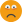I tried in a simple model with a
X=if(Value=0,0,100/Value)
onClick : ANIMATESLOW(Value,100,0)

The component must move from X=1 to X=infinite, but must finish at X=0
But when the value is 0 there is an error and the component stop where is it.
I think if there is an error, SU don't interpret nothing0DareDevil

### Re: My week-end challenge

Did you try the whole thing for AngleA and AngleB as I set it out ?
Perhaps putting ( ) around the second action...
0
TIGTIG
Global Moderator

### Re: My week-end challenge

Not needed to touch the AngleA and AngleB, the problem is not there because the Course never be 0 (Course is the total length of each the hydraulic cylinder, not yet draw, and it never be equal to zero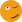)
The problem is in the Verin X and Z calculated with the formulas
X = L*(sin((copy+.5)*A)-sin(A/2))/(2*sin(A/2))
Z = L*(cos(A/2)-cos((copy+.5)*A))/(2*sin(A/2))

The L is the length of a pieces of the bridge floor and A is the angle between two pieces, so it will be equal to zero when the bridge is in the final position.
These formulas are equivalent to
X = L*(cos(A)+cos(2*A)+cos(3*A)+...+cos(n*A))
Z = L*(sin(A)+sin(2*A)+sin(3*A)+...+sin(n*A))

If there is an other equivalent without a division by sin(a) it will be nice...
0DareDevil

### Re: My week-end challenge

X = IF(sin(A/2)=0, 0, L*(sin((copy+.5)*A)-sin(A/2))/(2*sin(A/2)))
???
0
TIGTIG
Global Moderator

### Re: My week-end challenge

X = IF(sin(A/2)=0, 0, L*(sin((copy+.5)*A)-sin(A/2))/(2*sin(A/2)))
???

NoAlways saying there is divided by zero and don't return the zero when A=0 (and so sin(A/2)=0)
0DareDevil

### Re: My week-end challenge

Finished !
Rolling_08.skp

I haven't solved the divide by zero problem... but it works, and slowly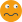0DareDevil

### Re: My week-end challenge

You deserve a medal for that! With a day to spare what will you do with your time?0
There's a frontroom and a backroom....reverse facesRich O Brien

### Re: My week-end challenge

Yes, well done!
An excellent 'complex DC'.

I still can't see why the IF() doesn't work to trap zero-divide issue ?
perhaps extra ( ) ???
X = IF((sin(A/2)=0), 0, (L*(sin((copy+.5)*A)-sin(A/2))/(2*sin(A/2))))
0
TIGTIG
Global Moderator

### Re: My week-end challenge

TIG wrote:I still can't see why the IF() doesn't work to trap zero-divide issue ?
perhaps extra ( ) ???
X = IF((sin(A/2)=0), 0, (L*(sin((copy+.5)*A)-sin(A/2))/(2*sin(A/2))))

Testing this kind of solution, I think SU want to evaluate any member of the IF before solving it, and if is an error it returns nothing but an error, even the error is in a non needed part of the functionBut...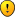Reading your post I had a machiavellian idea : two IF in the function, one like you propose, one under the divide
X = IF(sin(A/2)=0, 0, L*(sin((copy+.5)*A)-sin(A/2))/IF(2*sin(A/2)=0, 1, 2*sin(A/2))
The value for the true second IF can be anything but zero, just to avoid the divide by zero error, and the final result will be zero due to the first IF.

It works on the Divide_Zero.skp I sent before when Value = 0
X = if(Value=0,0,100/if(Value=0, 1, Value))

I will test it soon on the bridge model. It's already slow, and with 2 IF more in each part of the bridge, it can't be faster. But in real, it's slow0DareDevil

### Re: My week-end challenge

Rich O'Brien wrote:You deserve a medal for that! With a day to spare what will you do with your time?Looking for an other challenge !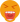0DareDevil

### Re: My week-end challenge

OR perhaps go back to where 'A' is set and use something like
=IF(A=0, 0.0001, A) then A is never quite 0 ?
0
TIGTIG
Global Moderator

### Re: My week-end challenge

TIG wrote:OR perhaps go back to where 'A' is set and use something like
=IF(A=0, 0.0001, A) then A is never quite 0 ?

Good idea too !!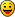Faster, but the bridge never be horizontal. OK, 0.0001 is quite horizontal... but not. I'm a man thinking Zero is not quite ZeroSo two solutions : one simple and faster but cant realy accept all angles, the other more mathematic but slow and made long sentences.

Remember me the time I worked as multimedia designer. Me and a friend worked on Flash and for the same job, I made 1 frame and 200 lines of actionscript, and my friend made 1 line of actionscript and 200 frames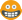0DareDevil

### Re: My week-end challenge

You'll also probably find that when it looks flat, in reality the bridge is slightly 'arched' [in a shallow 'hump'], because it will deflect under its own weight and the people standing on it, the engineer would want it to 'settle-down' to be no more than 'flat', in a 'worst case'.
So this is then even more complicated, because it means at some point the parts will go from a positive 'arch' to a negative 'curl', passing through 'zero' just after it has started to 'rollup'...0
TIGTIG
Global Moderator

### Re: My week-end challenge

TIG wrote:You'll also probably find that when it looks flat, in reality the bridge is slightly 'arched' [in a shallow 'hump'], because it will deflect under its own weight and the people standing on it, the engineer would want it to 'settle-down' to be no more than 'flat', in a 'worst case'.
So this is then even more complicated, because it means at some point the parts will go from a positive 'arch' to a negative 'curl', passing through 'zero' just after it has started to 'rollup'...Yes, I noticed the arc, but not at the end.
If you set the Course value to can se the end of the bridge dive into the pierIn the final position, Course = 109 (or 109.2 to avoid the divide by zero) all is quite horizontal in my model.
But in the model quite all is parametric. So if I want to have an arc at the end, just change the Lb value for little greater. This dimension is the size of the arms, that don't change the mesh size but just de calculation, so the axes aren't on the right place, but at the end the bridge is arched.
0DareDevil

### Re: My week-end challenge

Here is a little guide to understand the variables :
02_1.jpg

All the variables don't change the shape of the bridge, only the calculation.
Only Course, La and Lb are variables ; Angles are calculated with them.

0DareDevil

### Re: My week-end challenge

i had a similar problem before..

the way i ended up dealing with it was by adding another attribute.. it wasn't elegant at the time and it still isn't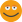..your double if statement seems better but i'll post the method anyway.. (might get the discussion going again to find a better solution than either of these)

for your Divide_Zero.skp, it would look like:

Position
X =if(trap=.12345,0,100/trap)

Custom
trap =if(value=0,.12345,value)
value =(user input)

you'd just have to make sure the trap value (.12345 in this example) is a number that should never come up as input..

not sure but doing it like this might speed things up if you have to enter the double IF statement for all parts of the bridge.. this will result in one IF statement in each part of the bridge plus a single extra IF
0
dotdotdotjeff hammond
Global Moderator

### Re: My week-end challenge

daredevil wrote:Here is a little guide to understand the variables :
02_1.jpg

All the variables don't change the shape of the bridge, only the calculation.
Only Course, La and Lb are variables ; Angles are calculated with them.

Instead of checking sin(a) have you considered checking the angle. There are only a few cases( maybe only a=0) that would need checking constrained by your geo. Conversely can't you create a variable say z= sin(a) and do a check on that?? you could even go as far as using a taylor series exapnsion for all if SU has problem with small angles.
0

mac1
Top SketchUcator

### Re: My week-end challenge

Jeff Hammond wrote:Position
X =if(trap=.12345,0,100/trap)

Custom
trap =if(value=0,.12345,value)
value =(user input)

I like this solutionSimple and economic.
As you say, the only inconvenient is to found an unusable value for the test. In the case of the bridge, no problem? because the angles are limited.
I will test it soon0DareDevil

### Re: My week-end challenge

Three years ago, I made this model to test DC and my trigonometry knowledge. I bring out it to show my students the wonder of SU Dynamic Components.
But...

After the divide by zero problem we talk in this thread, I have a new problem with SU 2015 (on Windows 7 and 8 64): it seems that there are any problems with very small numbers.

That's what happen when I roll and unroll the bridge on SU 2015 :
SU2015.jpg

That's is the good position after the sams operation on SU 8 :
SU8.jpg

If I explore components, I find it :
DC_SU2015.jpg

An error is highlighted on a very little number with a negative exponent e-6. Is the result of a COS calculated in the formula.
Perhaps SU DC don't understand this way to write a number and consider it like a text... Perhaps...

Some one have same issue with this model or other DC ?
0DareDevil